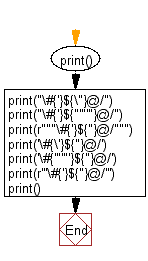﻿ Python: Define a string containing special characters in various forms - w3resource# Python: Define a string containing special characters in various forms

## Python Basic: Exercise-92 with Solution

Write a Python program to define a string containing special characters in various forms.

Sample Solution :-

Python Code :

``````print()
print("\#{'}\${\"}@/")
print("\#{'}\${"'"'"}@/")
print(r"""\#{'}\${"}@/""")
print('\#{\'}\${"}@/')
print('\#{'"'"'}\${"}@/')
print(r'''\#{'}\${"}@/''')
print()
```
```

Sample Output:

```\#{'}\${"}@/
\#{'}\${"}@/
\#{'}\${"}@/
\#{'}\${"}@/
\#{'}\${"}@/
\#{'}\${"}@/
```

Flowchart:## Visualize Python code execution:

The following tool visualize what the computer is doing step-by-step as it executes the said program:

Python Code Editor:

Have another way to solve this solution? Contribute your code (and comments) through Disqus.

What is the difficulty level of this exercise?

Test your Programming skills with w3resource's quiz.

﻿

## Python: Tips of the Day

Curries a function:

Example:

```from functools import partial

def tips_curry(fn, *args):
return partial(fn,*args)
add = lambda x, y: x + y
```100